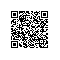# thinkphp中的AJAX返回ajaxReturn()

status 操作状态
info 提示信息
data 返回数据 
$this->ajaxReturn(返回数据,提示信息,操作状态); 返回数据data可以支持字符串、数字和数组、对象，返回客户端的时候根据不同的返回格式进行编码后传输。如果是JSON格式，会自动编码成JSON字符串，如果是XML方式，会自动编码成XML字符串，如果是EVAL方式的话，只会输出字符串data数据，并且忽略status和info信息。 下面是一个简单的例子： $User=M("User");//实例化User对象
$result =$User->add($data); if ($result){
//成功后返回客户端新增的用户ID，并返回提示信息和操作状态
$this->ajaxReturn($result,"新增成功！",1);
}else{
//错误后返回错误的操作状态和提示信息
$this->ajaxReturn(0,"新增错误！",0); } $data['status'] = 1;
$data['info'] = 'info';$data['size'] = 9;
$data['url'] =$url;
$this->ajaxReturn($data,'JSON');

thinkphp源代码：

/**
* Ajax方式返回数据到客户端
* @access protected
* @param mixed $data 要返回的数据 * @param String$type AJAX返回数据格式
* @return void
*/
protected function ajaxReturn($data,$type='') {
if(func_num_args()>2) {// 兼容3.0之前用法
$args = func_get_args(); array_shift($args);
$info = array();$info['data']   =   $data;$info['info']   =   array_shift($args);$info['status'] =   array_shift($args);$data           =   $info;$type           =   $args?array_shift($args):'';
}
if(empty($type))$type  =   C('DEFAULT_AJAX_RETURN');
if(strtoupper($type)=='JSON') { // 返回JSON数据格式到客户端 包含状态信息 header('Content-Type:text/html; charset=utf-8'); exit(json_encode($data));
}elseif(strtoupper($type)=='XML'){ // 返回xml格式数据 header('Content-Type:text/xml; charset=utf-8'); exit(xml_encode($data));
}elseif(strtoupper($type)=='EVAL'){ // 返回可执行的js脚本 header('Content-Type:text/html; charset=utf-8'); exit($data);
}else{
// TODO 增加其它格式
}
}使用钉钉扫一扫加入圈子
+ 订阅